Algebra II : Graphing Functions with Complex Numbers

Example Questions

Example Question #151 : Imaginary Numbers

Solve for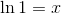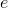Explanation:

Use the change of base formula for logarithmic functions and incorporate the fact that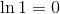and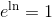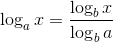Or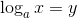can be solved using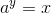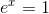Example Question #1 : Graphing Functions With Complex Numbers

Where would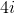fall on the number line?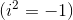Cannot be determined

to the left ofto the right ofatCannot be determined

Explanation:

Imaginary numbers do not fall on the number line-- they are by definition not real numbers.

** If the question asked where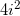falls on the number line, the answer would be to the left of 0, because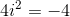.

Example Question #111 : Algebra

Write the complex number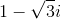in polar form, that is, in terms of a distance from the originon the complex plane and an angle from the positive-axis,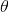, measured in radians.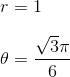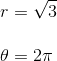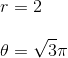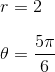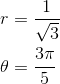Explanation:

To see what the polar form of the number is, it helps to draw it on a graph, where the horizontal axis is the imaginary part and the vertical axis the real part. This is called the complex plane.To find the angle, we can find its supplementary angle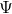and subtract it fromradians, so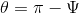.

Using trigonometric ratios,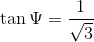and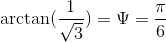.

Then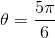.

To find the distance, we need to find the distance from the origin to the point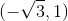. Using the Pythagorean Theorem to find the hypotenuse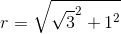or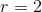.

All Algebra II Resources